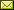## Quantum Probability as an Application of Data Compression Principles

 Allan F. Randall

 Realist, no-collapse interpretations of quantum mechanics, such as Everett's, face the probability problem: how to justify the norm-squared (Born) rule from the wavefunction alone. While any basis-independent measure can only be norm-squared (due to the Gleason-Busch Theorem) this fact conflicts with various popular, non-wavefunction-based phenomenological measures - such as observer, outcome or world counting - that are frequently demanded of Everettians. These alternatives conflict, however, with the wavefunction realism upon which Everett's approach rests, which seems to call for an objective, basis-independent measure based only on wavefunction amplitudes. The ability of quantum probabilities to destructively interfere with each other, however, makes it difficult to see how probabilities can be derived solely from amplitudes in an intuitively appealing way. I argue that the use of algorithmic probability can solve this problem, since the objective, single-case probability measure that wavefunction realism demands is exactly what algorithmic information theory was designed to provide. The result is an intuitive account of complex-valued amplitudes, as coefficients in an optimal lossy data compression, such that changes in algorithmic information content (entropy deltas) are associated with phenomenal transitions.

In Alastair A. Abbott and Dominic C. Horsman: Proceedings of the 7th International Workshop on Physics and Computation (PC 2016), Manchester, UK, 14 July 2016, Electronic Proceedings in Theoretical Computer Science 214, pp. 29–40.
Published: 21st June 2016.

 ArXived at: https://dx.doi.org/10.4204/EPTCS.214.6 bibtex PDF
References in reconstructed bibtex, XML and HTML format (approximated).
 Comments and questions to:eptcs@eptcs.org For website issues:webmaster@eptcs.org